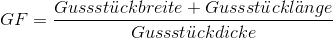## Shape factor

According to eq. 1, the shape factor SF is defined as the ratio of the length and width to the thickness of a casting.

Eq. 1:For compact castings, e.g. in the shape of a cube, sphere, ingot or plate, the modular ratio is a linear function of the casting’s shape factor SF, i.e. there is a linear dependence between the modular ratio (ratio of risermodulus Mr to casting modulus Mc) and the shape factor SF, as illustrated in Fig. 1.

The aim of this observation is to obtain correct dimensioning of risers since a riser can be sized somewhat smaller at a high modular ratio than at a low modular ratio, or in more precise words: As the shape factor SF rises, the modular ratio Mr/Mc increases. Therefore, castings having extensive surfaces such as plates or ingots require a high modular ratio at a relatively low riser volume, while compact castings such as spheres or cubes can be seal-fed with a smaller modular ratio and higher relative riser volume.